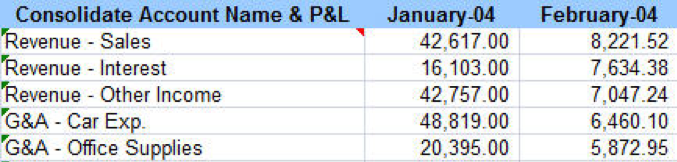Classes available in-person (strict social distancing) or live online with an extended free retake period. See details.# VLOOKUP Function in Excel

## Find Information Quickly and Efficiently Using VLOOKUP

VLOOKUP is an extremely useful function for finding information in one column and then returning information in a column to the right in the same row. Learn how to utilize Excel's VLOOKUP function to simplify your data search.

## Let's Look at an Example

VLOOKUP is often used to re-organize data that exists in a Pivot Table.

=VLOOKUP (Lookup value, Table Array, Column Index number, Range Lookup)

 Lookup Value: the cell that has the value being looked-up Table Array the cell array where the data is kept Column Number the number of the column in the array where the result will be found Range Lookup False (0) = exact match, True (1) = closet match, without going over

*NOTE: the Look-Up Value must be in the first column of Table Array

Example: We could “look up” Revenue – Sales” and have it return “February 2004” value of \$8,221.52### Things to remember when creating your VLOOKUP

• Your lookup value for your match and VLOOKUP is always different
• Your lookup table array is always “chunky”- it’s a contiguous block of text, starting with the column that your lookup value is found